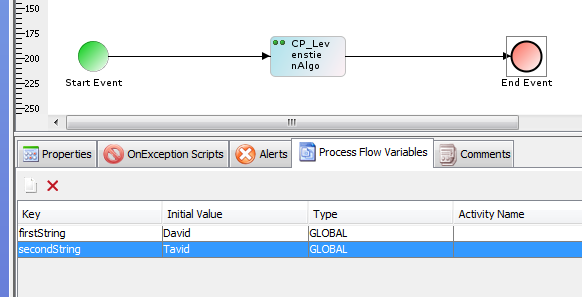# Levenshtein Algorithm (Fuzzy Matching)

Introduction

Levenshtein distance is a string metric for measuring the difference between two sequences. Informally, the Levenshtein distance between two words is equal to the number of single-character edits required to change one word into the other. The term edit distance is often used to refer specifically to Levenshtein distance.

The Levenshtein distance between two strings is defined as the minimum number of edits needed to transform one string into the other, with the allowable edit operations being insertion, deletion, or substitution of a single character.

For example, the Levenshtein distance between "kitten" and "sitting" is 3, since the following three edits change one into the other, and there is no way to do it with fewer than three edits:

1. kitten → sitten (substitution of 's' for 'k')

2. sitten → sittin (substitution of 'i' for 'e')

3. sittin → sitting (insertion of 'g' at the end).

We can use this plug-in in Adeptia process to find the Levenshtein distance between two stringsThe input of this plug-in will be 2 string values and will set the Levenshtein_distance variable in the process flow context which can be used further.